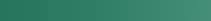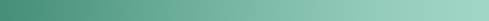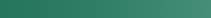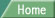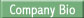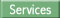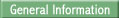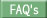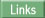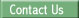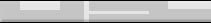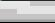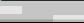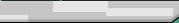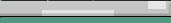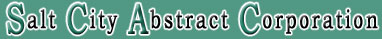Linear Measure

Square Measure1 inch = 0833 ft. 7.92 inches = 1 link 12 inches = 1 foot 1 vara = approx. 33 inches 2 3/4 feet = approve 1 vara 3 feet = 1 yard 25 links = 161/2 feet 100 links = 1 chain 161/2 feet = 1 rod 5 1/2 yards = 1 rod 4 rods = 100 links 66 feet = 1 mile 80 chains = 1 mile 320 rods = 1 mile 5280 feet = 1 mile 1760 yards = 1 mile

 144 sq. in = 1 sq. ft. 9 sq. feet = 1 sq. yard 30 1/4 sq. yds. = 1 sq. rod 16 sq. rods = 1 sq. chain q sq. rod = 272 1/4 1 sq. ch. = 4356 sq. ft. 10 sq. chs. = 1 acre 160 sq. rods = 1 acre 480 sq. yds. = 1 acre 43560 sq. ft. = 1 acre 640 acres = 1 sq. mile 1 section = 1 sq. mile 1 Twp. = 36 sq. miles 1 Twp. = 6 miles sq.